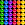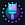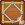# Multiply Ball - Puzzle Game

by AppRover
1,000,000+ installs

Android application Multiply Ball - Puzzle Game developed by is listed under category Game Puzzle. According to Google Play Multiply Ball - Puzzle Game achieved more than 1,000,000 installs. Multiply Ball - Puzzle Game currently has 14,438 ratings with average rating value of 4.34. The current percentage of ratings achieved in last 30 days is 0%, percentage of ratings achieved in last 60 days is 0%. Multiply Ball - Puzzle Game has the current market position #35962 by number of ratings. A sample of the market history data for Multiply Ball - Puzzle Game can be found below. Last update on 2023-09-02.

Title: Multiply Ball - Puzzle Game AppRover Game Puzzle Free Android
Total ratings: 14438 0% 0% 4.34
Installs (achieved): 1,000,000+ 1,000,000
5 star ratings: 10,667 893 1,340 268 1,250

2021-09-01: Android application Multiply Ball - Puzzle Game achieved 1,000,000 installs. Android application Multiply Ball - Puzzle Game achieved 500,000 installs. Android application Multiply Ball - Puzzle Game achieved 100,000 installs. Android application Multiply Ball - Puzzle Game achieved 10,000 installs.

Total number of ratings
Total number of active users rated for Multiply Ball - Puzzle Game.

Total number of installs (*estimated)
Estimation of total number of installs on Google Play. Approximated from number of ratings and install bounds achieved on Google Play.

Average rating
Average rating value on Google Play. Given by active users of the application.